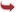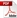International Journal of Scientific and Research Publications

#### IJSRP, Volume 4, Issue 11, November 2014 Edition [ISSN 2250-3153]Frequency response analysis of the gear box in a lathe machine using transfer functions
V Suma Priya, B V S Raghu Vamsi, E Kavitha, K. Srividya
Abstract: In this research work, frequency response of the gear box in the medium duty lathe machine is studied using transfer functions. The effect of the torque acting at a particular rotor on the amplitude of vibration of the other rotors is studied. Initially, equation of motion is developed for the multi-rotor system in the gearbox and later, Laplace transforms are applied to find the transfer functions. Torque acting at various rotors is also calculated. The obtained characteristic equation and the transfer functions are solved for poles and zeros (frequencies to attenuate the inputs at every rotor) by writing programming in MATLAB. Plots of the frequency response curves are plotted by writing the programming in MATLAB and the final conclusions are drawn.
[VIEW FULL PAPER][DOWNLOAD]

Reference this Research Paper (copy & paste below code):

V Suma Priya, B V S Raghu Vamsi, E Kavitha, K. Srividya (2018); Frequency response analysis of the gear box in a lathe machine using transfer functions; Int J Sci Res Publ 4(11) (ISSN: 2250-3153). http://www.ijsrp.org/research-paper-1114.php?rp=P353317